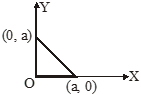Location of centre on mass for multiple point masses
Question

# Three rods of the same mass are placed as shown in the figure. What will be the co-ordinates of the center of mass of the system ?Easy
Solution

## Use the definition of C.M. : ${\mathrm{x}}_{\mathrm{cm}}=\frac{\sum {\mathrm{m}}_{\mathrm{i}}{\mathrm{x}}_{\mathrm{i}}}{\sum {\mathrm{m}}_{\mathrm{i}}}$

Get Instant Solutions